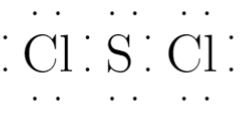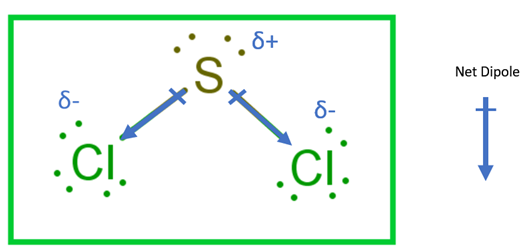# SCl2 Lewis Structure, Geometry, Hybridization, and PolaritySulfur dichloride is a red viscous liquid at room temperature. It has a pungent chlorine-like odor. It reacts with water to form chlorine-containing acids.

SCl2 is a very corrosive and toxic substance. It is a covalent compound because the electronegativity difference between S and Cl is not significant. They both are non-metals. S is in +2 oxidation state, and Cl is in -1 oxidation state.

It is soluble in hexane, benzene, carbon tetrachloride, etc.

SCl2 is unstable and exists in equilibrium with S2Cl2, as shown.SCl2 is used to synthesize other sulfur compounds like SOCl2, S4N4, S3H2, etc.

In this article, we will understand the concepts of Lewis dot structure, hybridization, and polarity.

We will also learn to determine the geometry and shape of a covalent compound using VSEPR theory.

Contents

## SCl2 Lewis Structure

Lewis Structure or Lewis dot structure is one of the basic methods to determine the type of bonds between atoms.

In this method, electrons in the valence shell are represented by dots, and two dots on different elements can be joined to form one bond.

It is a 2-D representation of bonding. It does not successfully explain all the properties but provides some vital information about the compound in focus.

Drawing of Lewis structure is a hit and trial method.

The final Lewis structure should satisfy the octet rule and formal charges. If it fails to satisfy either of them, another Lewis structure is drawn and checked for both.

### Octet Rule

The octet rule is followed by s and p block elements, collectively referred to as main group elements.

All the elements want to attain stability. The noble gases are considered inert and stable as they have a fully-filled configuration.

All the other elements prefer to make their valence shell completely filled with electrons to attain a noble gas-like configuration.

A fully filled valence shell configuration means that there are 8 electrons (like that of noble gas).

The ns and np subshells are completely filled, and hence it has 8 electrons. (2 from ns and 6 from np). The attainment of 8 electrons in the outermost shell fulfills the octet rule.

## Formal Charge

If the net charge on a compound is zero, it does not mean that charge in all the elements in that compound is zero.

A formal charge is the charge developed on a particular atom when the charge of the compound is distributed between the atoms.

It is a theoretical concept.

This is valuable in predicting the correct and lowest energy Lewis Structure. It is calculated using the formula:### Steps to draw Lewis Structure of SCl2

Step 1. Count the total number of valence shell electrons on the compound.

This is done by adding the valence shell electrons of all the constituent atoms.

If the compound is positively charged, we subtract the charge, and if it is negatively charged, we add the charge to the sum of valence shell electrons.

 Atom Atomic Number Group Number Valence electrons according to group number Electronic configuration  (E.C.) Valence shell from E.C. Valence electrons from E.C. One S 16 16 6 1s2 2s2 2p6 3s2  3p4 n=3 6 Two   Cl 17 17 7 1s2 2s2 2p6 3s2  3p5 n=3 7 Total number of valence shell electrons= 6 + (7*2) =20

The Lewis dot structure for S and Cl are as follows-Step 2. Choose a suitable central atom for the compound.

The central atom is the least electronegative one out of the constituent atoms.

The central atom is supposed to share its electron density with all other atoms.

If the central atom is more electronegative than the side atom, the central atom will not share the electron density, and sharing will not be facilitated.

Thus, S is the central atom for this compound.

Step 3. Draw a skeletal diagram.

In this step, we have to arrange the side atoms and central atoms suitably.Step 4. Arrange the valence electrons around the elemental symbols.

The total valence shell electrons (calculated in step 1) are placed according to bond formation.Step 5. Complete the octet of atoms by forming bonds.

Each Cl atom has seven valence electrons in the isolated state. It shares one electron with S to complete the octet.

S has six valence electrons in the isolated state.

It shares one electron from both Cl atoms to complete the octet.Step 6. Calculate the formal charge on all atoms.

The net charge on this compound is zero. Therefore, the sum of formal charge on three atoms should come out to be zero.

 Atom Total number of valence electrons in free atom Total number  of lone pairs (Total number  of bonding electrons)*0.5 Formal Charge S 6 2 8*0.5=4 6-2-4=0 Cl1 7 3 8*0.5=4 7-3-4=0 Cl2 7 3 8*0.5=4 7-3-4=0

Thus, the structure drawn in step 5 is the best Lewis structure for SCl2. For a better understanding, one can go through this video

## SCl2 Geometry

Geometry refers to the 3D arrangement of atoms and bonds in a compound. Some lone pairs can distort the geometry, and the distorted arrangement is referred to as shape.

Lewis Structure tells us about the type of bonds, but it fails to predict the geometry and shape.

The VSEPR theory has overcome this drawback for covalent compounds. VSEPR theory refers to the valence shell electron pair repulsion theory.

According to VSEPR theory-

• The valence electron pairs repel each other, and this leads to instability.

• To make the arrangement of the electrons stable, the repulsions between them have to be decreased.

• As a result, electrons align themselves so that the repulsion is the least, and the distance between them is maximum.

• The stable arrangement of the valence electron pairs of atoms helps in determining the molecular geometry.

Valence shell electrons involved in bonding are called bonding pairs of electrons (bp), and those valence shell electrons that are not involved in bonding are called lone pairs of electrons (lp).## How to Predict Geometry Using VSEPR

Step 1. Count the number of valence shell electrons on the central atom and let it be equal to M (arbitrary variable)

In the case of SCl2, the central atom is S. S has 6 valence electrons. (Shown in step1 of drawing lewis structure)
M=6

Step 2. Count the number of side atoms and let it be equal to N(arbitrary variable). In SCl2, there are 2 side atoms and N=2

Step 3. If the compound is charged, subtract the charge from N for the positively charged compound and add the charge to N for the negatively charged compound. In SCl2, there is no contribution of charge and N=6 only.

Step 4. Add the contribution of side atoms and charge to the contribution of the central atom, i.e., M+N.
For SCl2, M+N=8

Step 5. Divide M+N by 2 to find total electron pairs affecting the shape.
For SCl2, there are 4 electron pairs.

Step 6. Divide the total electron pairs as bonding and non-bonding. The bonding electron pair is equal to the number of side atoms.

For SCl2, there are 2 side atoms. Thus, there are 2 bonding pairs of electrons and 2 non-bonding pairs of electrons.

Using this information, we can predict geometry and shape using the following table.

Electron geometry is tetrahedral, and the shape is bent.## SCl2 Hybridization

Bonding in many polyatomic compounds cannot be explained by using atomic orbitals. For example- ammonia, water, etc.

Check out the hybridization of Ammonia.

The concept of hybridization can explain such compounds.

It is the mixing of pure atomic orbitals to form equivalent hybrid atomic orbitals. This mixing is feasible if the pure atomic orbitals have similar shapes and energy.

For example- The atomic orbitals of one 3s and two 3p can form three sp2 hybrid orbitals, but 3s and 7d cannot.S is the central atom in this compound.

The ground state electronic configuration of S is 1s2 2s2 2p6 3s2 3p4. Only valence orbitals are used in hybridization.There is no need for the promotion of electrons.

These 4 orbitals (one 3s and three 3p) undergo hybridization to form four sp3 orbitals, which will form bonds with the surrounding atoms.

In SCl2, there are 2 surrounding atoms. Each atom forms bond with one sp3 hybrid orbital.

The two sp3 hybrid orbitals have lone pair of electrons which do not participate in bonding.Two Cl-atoms form a sigma bond with sp3 hybrid orbitals. Thus, SCl2 has sp3 hybridization.

Another method for calculating the type of hybridization

In VSEPR theory, we calculated the total electron pairs in the last step. For SCl2, it came out to be 4.

From the table below, we can predict hybridization using total electron pairs or steric numbers.

The hybridization is sp3 for the SCl2 compound.## SCl2 Polarity

The polarity of a compound depends on the net dipole moment. The net dipole moment, in turn, depends mainly on-

• the difference in electronegativities of atoms forming bonds

• geometry of compound

In SCl2, only S-Cl bonds are present. The electronegativity of S and Cl is 2.58 and 3.16, respectively.

Due to this difference in electronegativity, S-Cl bonds are polar. The dipole moment vectors are directed from S to Cl.Now, the deciding factor is the shape of the compound.

If the shape were symmetrical, the dipole moment vectors would have canceled each other out, and the compound would have been non-polar.

But, the compound is bent and asymmetrical due to the presence of 2 lone pairs on S.

Hence, the dipole moment vectors do not cancel each other out, and hence the compound is polar.

BTW, I have also written an entire article on the polarity of SCl2. You must read out it once. check out the polarity of SCl2

## Conclusion

SCl2 is a covalent compound. It is liquid at room temperature.

The Lewis structure so formed satisfies the octet rule and formal charge. The electron geometry and shape are tetrahedral and bent, respectively. The hybridization is sp3.

It is a polar compound.

Happy Learning!